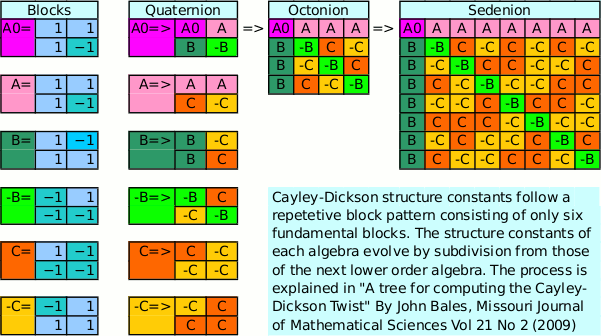# The Cayley-Dickson Calculator

## The universal real Cayley-Dickson algebra A is an infinite dimensional vector inner product space with Euclidean norm consisting of all sequences of real numbers of the form x0, x1,  . . .xn, 0, 0, 0,  . . . The canonical basis vectors for this space are denoted i0, i1, i2, i3,  . . . where i0 = 1, 0, 0, 0,  . . . i1 = 0, 1, 0, 0,  . . . i2 = 0, 0, 1, 0,  . . . i3 = 0, 0, 0, 1,  . . . etc Real numbers are identified with sequences of the form x0, 0, 0, 0,  . . . Complex numbers are identified with sequences of the form x0, x1, 0, 0, 0,  . . . Quaternions are identified with sequences of the form x0, x1, x2, x3, 0, 0, 0,  . . . Octonions are identified with sequences of the form x0, x1, x2, x3, x4, x5, x6, x7, 0, 0, 0,  . . . The Sedenions are identified with sequences of the form x0, x1,  . . .x15, 0, 0, 0,  . . . There is an infinite sequence of Cayley-Dickson algebras, each with twice the dimension of the previous algebra and each containing all previous algebras as proper sub-algebras. If the ordered pair (x, y) of two sequencesx = x0, x1, x2,  . . .andy = y0, y1, y2,  . . .is identified with the "shuffled" sequence (x, y) = x0, y0, x1, y1, x2, y2,  . . .then each algebra in the sequence beginning with the complex numbers consists of all ordered pairs of elements of the previous algebra.And for each element a,b ε A the ordered pair (a,b) ε A and if x ε A, then there are unique elements a,b ε A such that x = (a,b).The basis vectors obey the identities i2p = (ip, 0) i2p+1 = (0, ip) The conjugate of an element x = x0, x1,  . . .xn, 0, 0, 0,  . . . is the sequence x* = x0, –x1,  . . .–xn, 0, 0, 0,  . . . Thus for sequences x and y it follows that (x,y)* = (x*,–y).For sequences a, b, c and d for which multiplication is defined, the Cayley-Dickson product of the shuffled sequences (a,b) and (c,d) is defined by (a,b)·(c,d) = (ac-db*,a*d+cb).Using this product definition (there are alternate definitions), the product of the basis vectors is determined recursively as follows:If p and q are non-negative integers then i2p·i2q = (ip·iq, 0) i2p·i2q+1 = (0, ip*·iq) i2p+1·i2q = (0, iq·ip) i2p+1·i2q+1 =  –(iq·ip*, 0) The ultimate result of this recursive definition is a product of Cayley-Dickson basis vectors ip and iq given by the formula ip· iq = γ(p,q) ip^q where γ(p,q) is either +1 or -1 and where p^q is the bit-wise 'exclusive or' of the binary representations of p and q. [For example if p = 5=101B and q = 6=110B then p^q=101B^110B=011B=3. So i5·i6 =  γ(5,6)i3.]The sign function γ is called a "twist" and can be recursively defined as follows: γ(0,0) = γ(p,0) = γ(0,p) = 1 γ(2p,2q) = γ(p,q) γ(2p+1,2q) = γ(q,p) γ(2p,2q+1) = –γ(p,q) if p ≠ 0 and = 1 otherwise γ(2p+1,2q+1) = γ(q,p) if p ≠ 0 and = –1 otherwise The proof of the Cayley-Dickson twist is given in "Cayley-Dickson and Clifford Algebras as Twisted Group Algebras (2003)" by John W. Bales.You may use the following javascript applet to find the product of any two of the first 109-1 basis vectors. Larger values of p and q can trigger the sign bit when rendering the value of p^q. Enter a value of p: Enter a value of q: The value of p^q is: The value of γ(p,q) is: For the universal Cayley-Dickson algebra, if 0 ≠ p≠ q≠ 0 and if ip· iq = ir then iq· ip = –ir iq· ir = ip ir· ip = iq These may be called the "quaternion" properties. If 0 ≠ p≠ q≠ 0 and if ip· iq = ir then we denote this by the ordered number triple (p,q,r). For the universal Cayley-Dickson algebra (p,q,r) implies (q,r,p) and (r,p,q) by the quaternion properties, and by the properties of γ implies (2p,2q,2r), (2q,2p+1,2r+1), (2q+1,2p,2r+1) and (2q+1,2p+1,2r). Beginning with (1,2n,2n+1) for all n, these recursively generate the multiplication table for the universal Cayley-Dickson algebra when 0 ≠ p≠ q≠ 0. To complete the table one needs only to note that i0 = 1 and ip· ip = –1 for p ≠ 0.Summarizing, (1,2n,2n+1) is true for all n [The basis identity] and for 0 ≠ p≠ q≠ 0: (p,q,r) ⇒ (q,r,p) ⇒ (r,p,q) [The quaternion identities] (p,q,r) ⇒ (2p,2q,2r) (p,q,r) ⇒ (2q,2p+1,2r+1) (p,q,r) ⇒ (2q+1,2p,2r+1) (p,q,r) ⇒ (2q+1,2p+1,2r) The last four identities are the inductive identities and are implied by the nature of γ. The inductive identities are summarized in the following table: (p,q,r) 2p 2p+1 2q –2r 2r+1 2q+1 2r+1 2r [ Note to Octonion specialists: This indexing of the octonion basis vectors, automatically satisfies index doubling, but not index cycling. For more on this topic go here.] Given the Euclidean inner product <x,y>, the universal Cayley-Dickson algebra A satisfies the adjoint properties <x·y, z> = <x, z·y*> <x·y, z> = <y, x*·z> Thus the kth component of the product x·y, is <x·y, ik> = <x, ik·y*>.Also check out the Sedenion Reverse Polish Notation (RPN) Calculator,the Octonion RPN calculator,and the Sedenion Norm Comparison Calculator.And the Color-coded Clifford Algebra Multiplication TableReference "Cayley-Dickson and Clifford Algebras as Twisted Group Algebras" (by J. W. Bales (2003); Dept. of Mathematics, Tuskegee UniversityReference "Properly twisted groups and their algebras" by J. W. Bales (2006); Dept. of Mathematics, Tuskegee UniversityReference "A catalog of Cayley-Dickson-like products" by J. W. Bales (2011); Dept. of Mathematics, Tuskegee UniversityReference "A tree for computing the Cayley-Dickson twist" by J. W. Bales (2007) published in the Missouri Journal of Mathematical Sciences Vol. 21 No. 2 (2009)© John Wayland Bales (2009)Reference "The eight Cayley-Dickson doubling products" by J. W. Bales (2015); Advanced in Applied Clifford Algebras (Springer)

Links to other content of this web domain for the benefit of web crawlers: